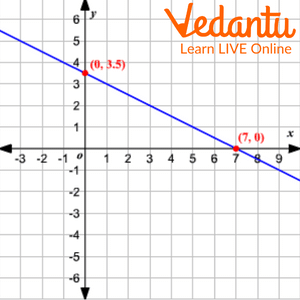Courses
Courses for Kids
Free study material
Free LIVE classes
More

# Intercepts of a LineLIVE
Join Vedantu’s FREE Mastercalss

## What is the Intercept of a Line?

The x-intercept and the y-intercept are the two different types of intercepts. The line's actual point of intersection with the x-axis is known as the x-intercept, while its actual point of intersection with the y-axis is known as the y-intercept. In this article, we will define the intercept, show how to obtain the intercept for a given line, and demonstrate how to graph intercepts.

## What is x-Intercept?

The general form of the linear equation is written as $y = mx + c$, where m and c are constants. The line's crossing point, which is located at the x-axis of the plane, is known as the x-intercept. This indicates that anytime a linear equation crosses the x-axis, its y-coordinate value will always be equal to 0. The y-coordinate is zero for the x-intercept and the x-coordinate is zero for the y-intercept. The term "horizontal intercept" also applies to the x-intercept.

## What is y- Intercept?

The graph's intersection with the y-axis is known as the y-intercept. Finding the intercepts for any function with the formula $y = f\left( x \right)$ is crucial when graphing the function. An intercept can be one of two different forms for a function. The x-intercept and the y-intercept are what they are. A function's intercept is the location on the axis where the function's graph crosses it.

## Equation of a Line with Intercepts

How to find an intercept of a straight line? Intercepts are subject to various equations and formulas. By solving for x and putting $y = 0$ in the equation, all of the formulas are obtained.

Following are the steps to determine the y intercept of a function, where$y = f\left( x \right)$, where $x = 0$ is simply substituted.

Resolve for y.

Put the y-point intercepts in place (0, y).

## Conclusion

The two different kinds of intercepts are the x-intercept and the y-intercept. The x-intercept of a line is its actual point of intersection with the x-axis, and the y-intercept is its true point of intersection with the y-axis.

## Solved Examples

Example 1:Find the value of "a" if the y-intercept of a function $y = a(x - 1)\left( {x - 2} \right)\left( {x - 3} \right)$ is $\left( {0,12} \right)$ .

Ans: The given function's equation is:

$y = a(x - 1)\left( {x - 2} \right)\left( {x - 3} \right)$

By adding $x = 0$ to the y-intercept formula, it can be calculated.

$y = a \left( {0 - 1} \right]\left[ {0 - 2} \right]\left[ {0 - 3} \right]$ $= - 6a$

The y-intercept is therefore $\left( {0, - 6a} \right)$

However, the issue claims that the provided function's y-intercept is $\left( {0,12} \right)$. Thus,

$- 6a = 12$

Using -6 to divide both sides,

$a = - 2$

Example 2: Find the x and y intercept in the given graph.

##Ans: The x-intercept is 7 and y – intercept is 3.5 in the given graph.Example 3: Find the x-intercept of $y = 4x - 8$.Ans: For finding the x – intercept we must put y coordinate as 0.Thus, putting$y = 0$ ,we will get:$\begin{array}{l}0 = 4x - 8\\8 = 4x\\x = 2\end{array}$Therefore, x coordinate is 2.

Last updated date: 27th Sep 2023
Total views: 82.2k
Views today: 2.82k

## FAQs on Intercepts of a Line

1. What are the Y Intercept Formula Applications?

To determine a function's y-intercept, use the y-intercept formula. The procedure of graphing a function is when the y-intercept is most frequently employed.

2. Is 0 a possible x-Intercept?

For the line$y = mx$, where m is the line's slope and the value of the x-intercept is equal to 0, 0 can indeed serve as the x-intercept.

3. What shape does the value angle between the line's Y and X intercepts take if they are equal?

When a line's x-intercept and y-intercept are equal, the line intersects the x-axis at a 45° angle if we are taking in first quadrant.

4. How many intercepts are permitted on a line?

X-intercept and Y-intercept are the two main intercepts. The line's x-intercept and y-intercept are located where the line crosses the x and y axes, respectively.